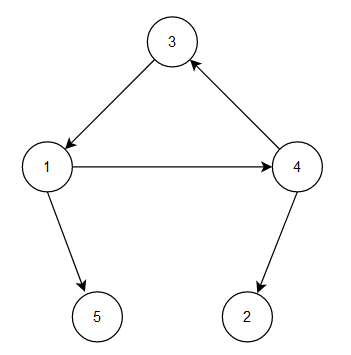# Queen's Palace

Posted: 25 Dec, 2020
Difficulty: Moderate

## PROBLEM STATEMENT

#### Can you find such a state where the queen can have her palace?

##### Example:
``````Let’s take an example for the below given Ninjaland.
````````````For the given example, the states from which we can reach all other states are 1, 3, 4. So, you can answer any one of them.
``````
##### Input format :
``````The first line contains an integer 'T' denoting the number of test cases.

The first line of each test case contains two space-separated integers 'N' and ‘M’ representing the number of states and number of roads in the country, respectively.

The next 'M' lines of every test case contain two single space-separated integers ‘A’ and ‘B’, representing a road between the states 'A', 'B' and directed from state 'A' to state 'B'.
``````
##### Output format:
``````For each test case, return a single integer denoting the state in which the queen should live. If there is no state from which the queen can reach all other states then return -1.
``````
##### Note:
``````You don't need to print anything, it has already been taken care of. Just implement the given function.
``````
##### Constraints:
``````1 <= T <= 100
1 <= N <= 1000
1 <= M <= 2000

Time limit: 1 sec
``````Approach 1

The very first approach can be to try all states and check if we can reach all other states from the current state.

The steps are as follows:

• Select each state for the queen.
• Try to visit all possible states from the selected state using a DFS or BFS.
• If the count of visited states is equal to the total number of states then the queen can live in the selected state.